Next: Exercises Up: Coupled Oscillations Previous: Spring-Coupled Masses

# Triatomic Molecule

Consider the simple model of a linear triatomic molecule (e.g., carbon dioxide) illustrated in Figure 38. The molecule consists of a central atom of massflanked by two identical atoms of mass. The atomic bonds are represented as springs of spring constant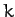. The linear displacements of the flanking atoms are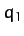and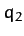, whilst that of the central atom is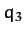. Let us investigate the linear modes of oscillation our model molecule.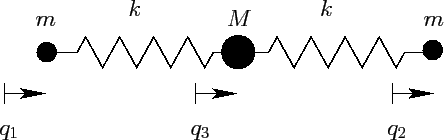The kinetic energy of the molecule is written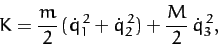(840)

whereas the potential energy takes the form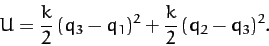(841)

Clearly, we have a three degree of freedom dynamical system. However, we can reduce this to a two degree of freedom system by only considering oscillatory modes of motion, and, hence, neglecting translational modes. We can achieve this by demanding that the center of mass of the system remains stationary. In other words, we require that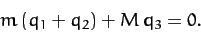(842)

This constraint can be rearranged to give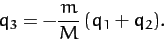(843)

Eliminatingfrom Equations (840) and (841), we obtain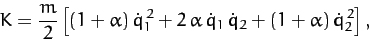(844)

and(845)

respectively, where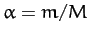.

A comparison of the above expressions with the standard forms (775) and (780) yields the following expressions for the mass matrix,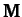, and the force matrix,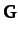: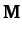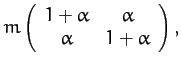(846)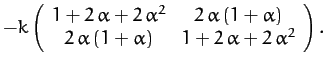(847)

Now, the equation of motion of the system takes the form [see Equation (786)]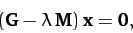(848)

where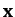is the column vector of theandvalues. The solubility condition for the above equation is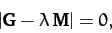(849)

which yields the following quadratic equation for the eigenvalue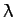: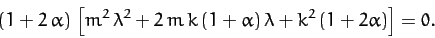(850)

The two roots of the above equation are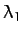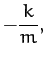(851)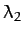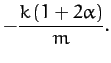(852)

The fact that the roots are negative implies that both normal modes are indeed oscillatory in nature. The characteristic oscillation frequencies are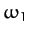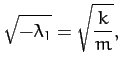(853)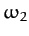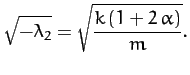(854)

Equation (848) can now be solved, subject to the normalization condition (799), to give the two eigenvectors: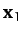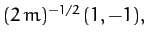(855)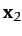(856)

Thus, we conclude from Equations (843) and (853)-(856) that our model molecule possesses two normal modes of oscillation. The first mode oscillates at the frequency, and is an anti-symmetric mode in which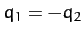and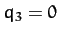. In other words, in this mode of oscillation, the two end atoms move in opposite directions whilst the central atom remains stationary. The second mode oscillates at the frequency, and is a mixed symmetry mode in which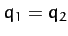but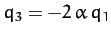. In other words, in this mode of oscillation, the two end atoms move in the same direction whilst the central atom moves in the opposite direction.

Finally, it is easily demonstrated that the normal coordinates of the system are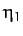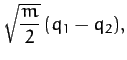(857)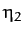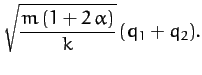(858)

When expressed in terms of these coordinates,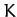andreduce to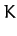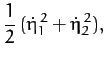(859)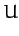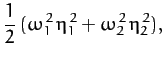(860)

respectively.Next: Exercises Up: Coupled Oscillations Previous: Spring-Coupled Masses
Richard Fitzpatrick 2011-03-31